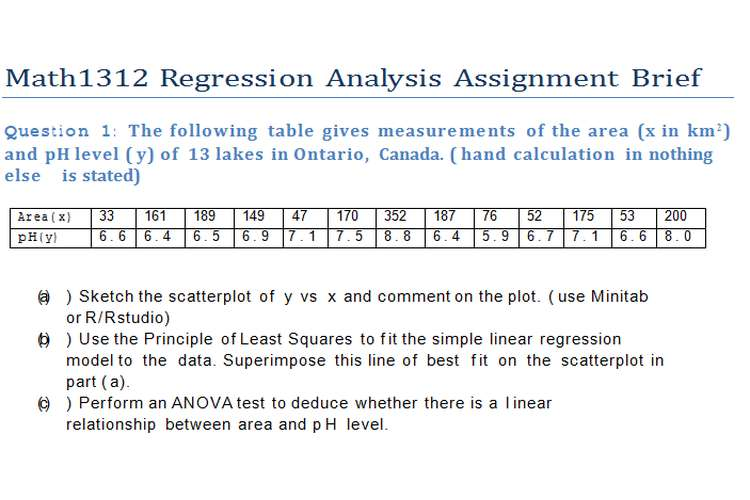Math1312 Regression Analysis Editing and Paper Editing ServicesQuestion 1: The  following  table  gives  measurements  of  the  area  ( x  in  km 2 )  and  p H level ( y) of  13 lakes in Ontario, Canada. ( hand calculation in nothing else    is stated)

 Ar ea( x) 33 161 189 149 47 170 352 187 76 52 175 53 200 p H( y) 6 . 6 6 . 4 6 . 5 6 . 9 7 . 1 7 . 5 8 . 8 6 . 4 5 . 9 6 . 7 7 . 1 6 . 6 8 . 0
1. )  Sketch the scatterplot of  y  vs  x  and comment on the plot. ( use Minitab  or R/ Rstudio)
2. )  Use the Principle of Least Squares to f it the simple linear regression    model to  the  data. Superimpose this line of  best  f it  on  the  scatterplot in part ( a).
3. )  Perform an ANOVA test to deduce whether there is a  l inear   relationship between area and p H  level.
4. )  Perform all appropriate residual checks using R/ Rstudio or  MINITAB  and clearly explain if  any of  the model assumptions have been   violated.
5. )  Another lake in the same region was found to have an area of  2050 km 2. Predict its  p H  level and f ind  a  99%  confidence interval of  this prediction.

( 3 + 8+ 5 + 8+ 3 = 27)

Question 2: ( Everything  to  be  done  by  hand  expect  is  otherwise  is  stated) The following data are  provided:

 a/a 1 2 3 4 5 6 7 8 9 10 11 12 13 X 35.3 29.7 30.8 58.8 61.4 71.3 74.4 76.7 70.7 57.5 46.4 28.9 28.1 Y 10.98 11.13 12.51 8.4 9.27 8.73 6.36 8.5 7.82 9.14 8.24 12.19 11.88 a/a 14 15 16 17 18 19 20 21 22 23 24 25 X 39.1 46.8 48.5 59.3 70 70 74.5 72.1 58.1 44.6 33.4 28.6 Y 9.57 10.94 9.58 10.09 8.11 6.83 8.88 7.68 8.47 8.86 10.36 11.08

Where X represent the steam in pounds per months and Y is the mean atmosphere temperature measured in  Fahrenheit.
Calculate the followings:

1. Fit a l inear regression model and give the least square estimates for the constant and the  slope.
2. Calculate the residuals for each of  the 25   observations.
3. Make the ANOVA table and complete it by performing all the required calculations. W hat is  the ANOVA table used  for?
4. Find the coefficient of determination and the correlation coefficient. Explain its value.
5. Calculate the std for the error, the std for the slope and the std for the constant.
6. Test whether the slope and the constant are significant. State the  needed hypotheses and explain your  results.
7. Construct the confidence intervals for the slope and for the constant.

( 8 + 4+ 6 + 4+ 6 + 4+ 6 = 38 )

Question 3: For  the  data  provided  in  the  above  question  2  do  the  following  using a statistical software ( either  Minitab  or  R/ Rstudio  will  do).  Include  the  used  code  for  R  or describe the steps in  details for  Minitab.

1. Generate a  scatterplot of  the data and comment on  it .
2. Answer all the queries a)- g) of Question 2 and comment on the derived outputs.
3. Using the generated residuals, test the assumption behind the simple linear regression.

( 2 + 26+ 7= 35)